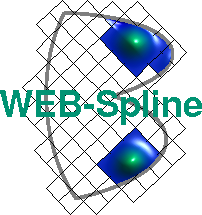# Helmholtz Equation

The group of the physicist Prof. Dr. A. Richter uses experiments with microwaves to study quantum manifestation of classical chaos. To this end, they build super-conductive microwave resonators and measure the spectrum of eigenvalues. For details click here.

As an example the following picture shows a flat resonator for a Pascalian snail: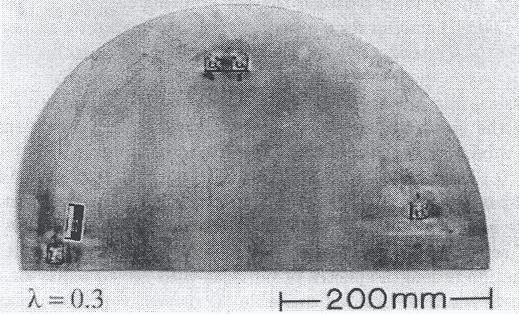The physical experiment is mathematically described by the stationary Helmholtz equation: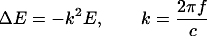The finite element discretisation of this equation leads to a generalised eigenvalue problem: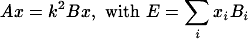Using Web-Splines of degree 5 on a 75x75 grid we are able to compute 2000 eigenvalues with relative error less then 10-4 in half an hour on a 2 GHz standard PC .

Here are some examples of the corresponding eigenfunctions:

first eigenfunction, k2 = 106.6774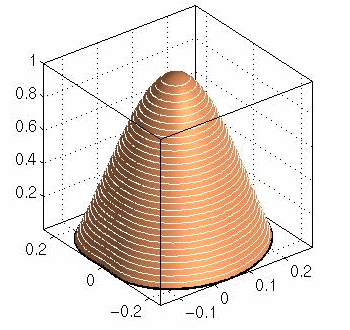second eigenfunction, k2 = 254.2339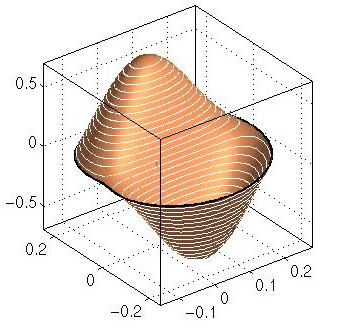third eigenfunction, k2 = 286.0975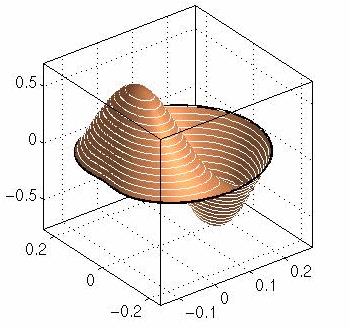tenth eigenfunction, k2 = 960.0726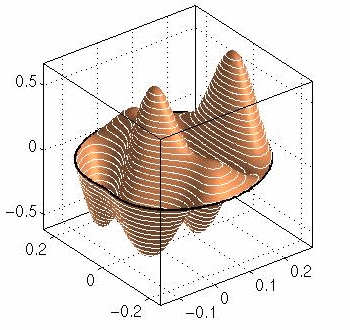fiftieth eigenfunction, k2 = 4119.0409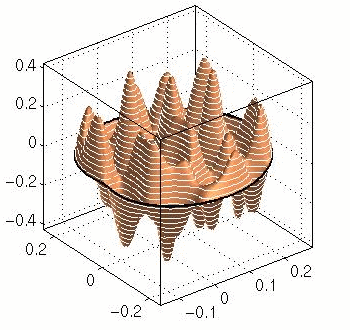### Literature

A. Richter: Playing Billiards with Microwaves - Quantum Manifestations of Classical Chaos, The IMA Volumes in Mathematics and its Application, Vol. 109, Springer 1999.

See Publications for a complete list of WEB-publications.

Author: Bernhard Mößner ; Last modification: 2003/10/17 16:17:04 UTC.

 [Home] [The WEB-Method] [Examples] [Publications] [Contact]